# Circle Problem: Solve an angle

I could.In summary, the figure is not symmetric, and to solve for z, you need to find the angles of the green and gray triangles, and also the sum x+y.

## Homework Statement[/B]

imgur.com/a/bsAKl

[I didn't know how else to upload an image from my iPad]

In this problem:
-Center of the circle=Point F
-Arc AB= 110 degrees
-Arc CD= 40 degrees

Find the measure of angle E.

## Homework Equations

I know of some equations, but I don't know if they apply to this particular problem.

## The Attempt at a Solution

I tried for about 10 minutes, but I didn't know where to begin. I was absent on the day this was taught (I think) and I don't feel like searching for this in the eBook.

Anyways, so far I know:
-Arc AB + Arc CD + something = 360 degrees

Other than that, I really don't know what else I can do with this info.

*I know the answer to this is 40 degrees because it was posted later, but I don't know how that is the answer.
My friend said that whenever he sees a triangle, he does the Triangle Angle Sum Theorem. He said he did 180 degrees - (110 degrees + 40 degrees) to get 40 degrees.
*I know that's incorrect because:
(A) That "shape" is not a triangle because AB and CD are arcs, not straight lines.
(B) The measurements are for the arcs, not the angles of the supposed "triangle". Arc =/= Angle.

BUT he still got it correct. A bunch of other people did that wrong method but got the correct answer as well, while I left it blank. Please help guys because this is going to be on the state final as well.

## Homework Statement[/B]

imgur.com/a/bsAKl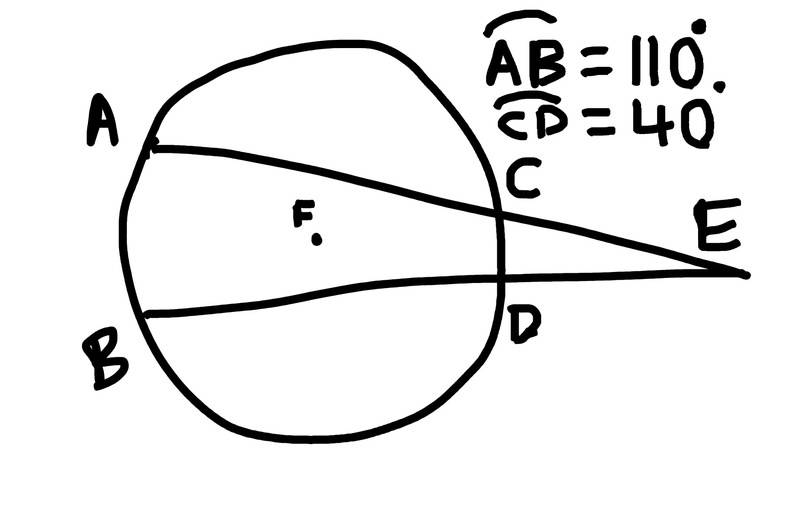In this problem:
-Center of the circle=Point F
-Arc AB= 110 degrees
-Arc CD= 40 degrees

Find the measure of angle E.

## Homework Equations

I know of some equations, but I don't know if they apply to this particular problem.

## The Attempt at a Solution

I tried for about 10 minutes, but I didn't know where to begin. I was absent on the day this was taught (I think) and I don't feel like searching for this in the eBook.

Anyways, so far I know:
-Arc AB + Arc CD + something = 360 degrees.
Why is the minus in front of Arc AB? And how much is that "something" then? what does it represent?

Other than that, I really don't know what else I can do with this info.

*I know the answer to this is 40 degrees because it was posted later, but I don't know how that is the answer.
My friend said that whenever he sees a triangle, he does the Triangle Angle Sum Theorem. He said he did 180 degrees - (110 degrees + 40 degrees) to get 40 degrees.
*I know that's incorrect because:
(A) That "shape" is not a triangle because AB and CD are arcs, not straight lines.
(B) The measurements are for the arcs, not the angles of the supposed "triangle". Arc =/= Angle.

BUT he still got it correct. A bunch of other people did that wrong method but got the correct answer as well, while I left it blank. Please help guys because this is going to be on the state final as well.
Well, it is utterly incorrect. The method is wrong, as you said. And 180-(110+40) is not 40!
Look at the picture. What are the angles x?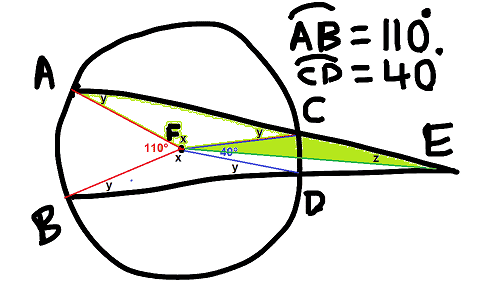In the triangles FAC and FBC, what are the angles y?
The line FE halves both the 40 angle and the angle at E. From the green triangle, what do you get for z?

Sorry for late response. I didn't mean negative arc, I didn't mean to use "-" as a minus sign, but rather a bullet point. Sorry for the confusion. "Something" is the measures of the other two arcs whose measures are not given. (Arc AC and Arc BD).

Also, I just remembered. In the original problem, Arc AB is a 100 degrees.

Okay, now for the solution, assuming arc AB is 100 degrees.

Now I am a little confused with your labelling. Why are angles AFC and BFD? Why are angles FAC and angles FBD equal?

Now I am a little confused with your labelling. Why are angles AFC and BFD? Why are angles FAC and angles FBD equal?
You are right, they should not be equal unless it is said so. What was written in the text of the problem?
The figure looked symmetric.

Last edited:
If the figure is not symmetric, it can look like the following picture: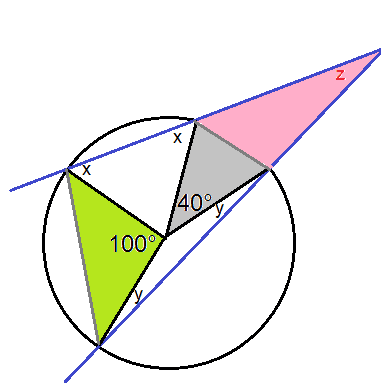You can determine the angles of the green and gray triangles, and also the sum x+y. With those, you get z, the angle in question.

ehild said:
If the figure is not symmetric, it can look like the following picture:
View attachment 205213

You can determine the angles of the green and gray triangles, and also the sum x+y. With those, you get z, the angle in question.
Hmm... I don't remember the original problem because I don't have a copy of it. I jotted down the picture from my memory. Anyways, I looked up this, and I found this on a website. It was hard for me to understand off of it, but I think that is what I am looking for.
http://www.mathopenref.com/secantangles.html

It's gives the theorem, but I want to know how to solve it without plugging it in the formula.

Hmm... I don't remember the original problem because I don't have a copy of it. I jotted down the picture from my memory. Anyways, I looked up this, and I found this on a website. It was hard for me to understand off of it, but I think that is what I am looking for.
http://www.mathopenref.com/secantangles.html

It's gives the theorem, but I want to know how to solve it without plugging it in the formula.
There are a few triangles on the picture in my previous post. You do not need anything else, but "the sum of angles of any triangle is 180°."
What are the central angles in the white triangles in terms of x and y? Note that the triangles are isosceles. So what is x+y?
What are the angles at A and B in the green triangle?
What are the angles at A and B in the big triangle ABE? So what is the angle at E?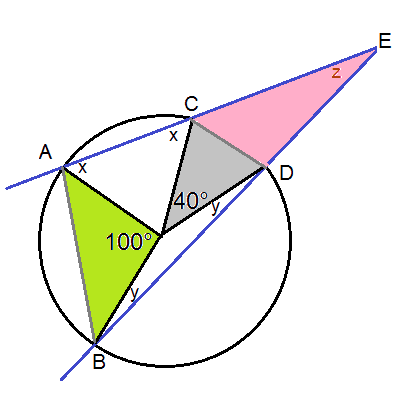ehild said:
There are a few triangles on the picture in my previous post. You do not need anything else, but "the sum of angles of any triangle is 180°."
What are the central angles in the white triangles in terms of x and y? Note that the triangles are isosceles. So what is x+y?
What are the angles at A and B in the green triangle?
What are the angles at A and B in the big triangle ABE? So what is the angle at E?
View attachment 205221
Oh. I see it now. But before I continue to solve it, why are the triangles isosceles?

Oh. I see it now. But before I
continue to solve it, why are the triangles isosceles?
OA, OB, OC, OD are radii of the circle.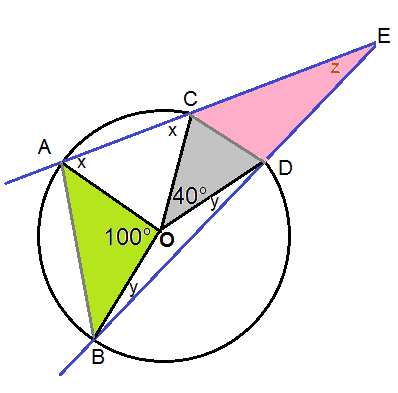•ehild said:
OA, OB, OC, OD are radii of the circle.
View attachment 205229
OMG, how could I overlook that. I feel stupid now. But thanks, I will attempt to solve it now, now that I know the 4 triangles are isosceles.
Okay...

Green Triangle
Base angles= [(180 degrees -100 degrees)/2] = 40 degrees
Grey Triangle
Base angles= [(180 degrees - 40 degrees)/2] = 70 degrees

So now, I don't know how to figure out the central angles of triangles AOC and BOD. How do I figure those out?

OMG, how could I overlook that. I feel stupid now. But thanks, I will attempt to solve it now, now that I know the 4 triangles are isosceles.
Okay...

Green Triangle
Base angles= [(180 degrees -100 degrees)/2] = 40 degrees
Grey Triangle
Base angles= [(180 degrees - 40 degrees)/2] = 70 degrees

So now, I don't know how to figure out the central angles of triangles AOC and BOD. How do I figure those out?
You can not. But you know the sum of those angles. And write out the relation between the central angles and the base angles x or y, and then you can figure out the sum x+y.

ehild said:
You can not. But you know the sum of those angles. And write out the relation between the central angles and the base angles x or y, and then you can figure out the sum x+y.
Sorry, I do not get how to write out the relationship between the central angles of the white triangles and x and y.

This might be wrong, but I can only figure out these relations.

180 degrees= [Angle BOD] + 2y
180 degrees= [Angle AOC] + 2x
360 degrees= 140 degrees + [Angle AOC] + [Angle BOD]
220 degrees= [Angle AOC] + [Angle BOD]
360 degrees= 220 degrees + 2y + 2x

Is there any critical reasoning/info I am overlooking/missing?

Sorry, I do not get how to write out the relationship between the central angles of the white triangles and x and y.

This might be wrong, but I can only figure out these relations.

180 degrees= [Angle BOD] + 2y
180 degrees= [Angle AOC] + 2x
360 degrees= 140 degrees + [Angle AOC] + [Angle BOD]
220 degrees= [Angle AOC] + [Angle BOD]
360 degrees= 220 degrees + 2y + 2x

Is there any critical reasoning/info I am overlooking/missing?
It is all right. So what is x+y?

Now look at the triangle ABE.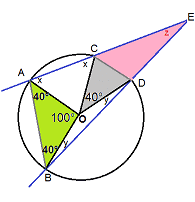The angle BAE ix 40°+x. The angle ABE is 40°+y. Write up the sum of the angles of triangle ABE, Substitute the value of x+y. What do you get for z?

ehild said:
It is all right. So what is x+y?

Now look at the triangle ABE.
View attachment 205283

The angle BAE ix 40°+x. The angle ABE is 40°+y. Write up the sum of the angles of triangle ABE, Substitute the value of x+y. What do you get for z?
Okay. So:

180 degrees= (40 degrees + x) + (40 degrees + y) + (Z)
180 degrees= 80 degrees + (x+y) +z

How do I figure out the value of "x+y"? That's where I am having trouble.

Okay. So:

180 degrees= (40 degrees + x) + (40 degrees + y) + (Z)
180 degrees= 80 degrees + (x+y) +z

How do I figure out the value of "x+y"? That's where I am having trouble.
You wrote in Post#12 that "360 degrees= 220 degrees + 2y + 2x". What is 2x+2y? what is x+y then?

ehild said:
You wrote in Post#12 that "360 degrees= 220 degrees + 2y + 2x". What is 2x+2y? what is x+y then?
You mean what it is? When I try to solve it through substitution method?

You mean what it is? When I try to solve it through substitution method?
I asked what is the numerical value of (2x+2y) if 360 =220 + 2x+2y.
Think: You add a number to 220 and you get 360. What number did you add?

ehild said:
I asked what is the numerical value of (2x+2y) if 360 =220 + 2x+2y.
Think: You add a number to 220 and you get 360. What number did you add?
Yes I see what you mean, let me explain.
I know that:
(2x+2y)=140 degrees

However, I tried to solve it fully a couple days ago, and I got infinite many solutions. But for your answer, 140 degrees.

EDIT:
I just thought of something. Could I simply:
2x+2y=140 degrees
To
1x+1y=70 degrees

By dividing by two? Is that what you meant?

Yes I see what you mean, let me explain.
I know that:
(2x+2y)=140 degrees

However, I tried to solve it fully a couple days ago, and I got infinite many solutions. But for your answer, 140 degrees.

EDIT:
I just thought of something. Could I simply:
2x+2y=140 degrees
To
1x+1y=70 degrees

By dividing by two? Is that what you meant?

Yes, it is. 2x+2y can be written as 2(x+y), by factoring out 2.
So 2(x+y)=140
dividing the equation by 2 : x+y=70°

•ehild said:
Yes, it is. 2x+2y can be written as 2(x+y), by factoring out 2.
So 2(x+y)=140
dividing the equation by 2 : x+y=70°
Nice! So now, back to the main problem.
180 degrees= z + x + (40 degrees) + (40 degrees) + y = (80 degrees) + (x+y) + z = 150 degrees + z

Solve:
180 degrees = 150 degrees + z
z = 30 degrees

z = Angle E

Angle E = 30 degrees

Is everything correct?

*If it is, then I guess the problem was 110 degrees for arc AB instead of 100 degrees, which would produce Angle E as 40 degrees, which was the answer.

Nice! So now, back to the main problem.
180 degrees= z + x + (40 degrees) + (40 degrees) + y = (80 degrees) + (x+y) + z = 150 degrees + z

Solve:
180 degrees = 150 degrees + z
z = 30 degrees

z = Angle E

Angle E = 30 degrees

Is everything correct?

*If it is, then I guess the problem was 110 degrees for arc AB instead of 100 degrees, which would produce Angle E as 40 degrees, which was the answer.
Yes, it is correct. Good work!
If arc AB was 110, ( x+y) would also change and z would not become 40°. For a quick check, see http://www.mathopenref.com/secantangles.html z would be 35°.

Last edited:
•You are welcome